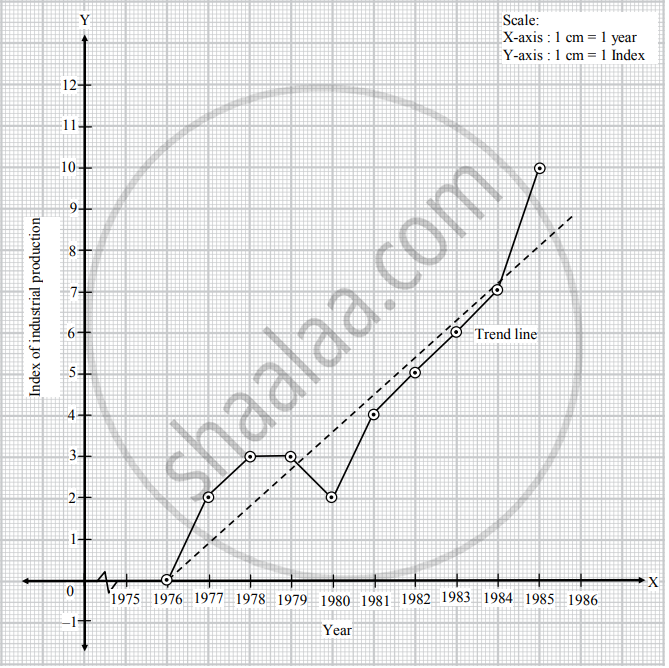# The following table shows the index of industrial production for the period from 1976 to 1985, using the year 1976 as the base year.Fit a trend line to the above data by graphical method. - Mathematics and Statistics

Graph

The following table shows the index of industrial production for the period from 1976 to 1985, using the year 1976 as the base year.

 Year 1976 1977 1978 1979 1980 1981 1982 1983 1984 1985 Index 0 2 3 3 2 4 5 6 7 10

Fit a trend line to the above data by graphical method.

#### Solution

Taking year on X-axis and index on Y-axis. We plot the points for indices corresponding to the years. Joining these points we get the graph of the given time series. We draw trend line as shown in the figure.Concept: Uses of Time Series Analysis
Is there an error in this question or solution?
Chapter 4: Time Series - Exercise 4.1 [Page 66]

Share